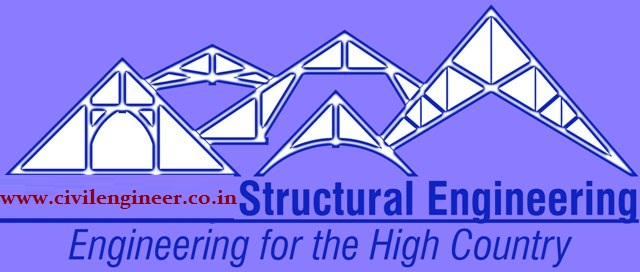# STRUCTURAL ENGINEERING- II

## 2013 (A) Subject Code : 101401

1.  Analyse the given beam  using moment distribution method :2. Analyse the given frame using moment distribution method :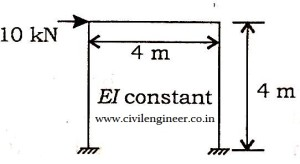3.  Analyse the given rigid frame using slope deflection method :4.  Analyse the given portal frame using slope deflection method :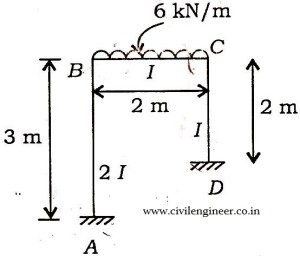5.  A two hinged parabolic arch has a span of 30 m and a central rise of 5 m. calculate the maximum positive and negative bending moment at a section distant 10 m from the left support, due to single point load of 10 KN rolling from left to right .

6. A Suspension bridge with two-hinged stiffening girder has a span of 100 m and the cables have a central dip of 10 m. The Stiffening girders are 4 m deep and have moment of inertia equal to 1.64 x 10^10 mm^4. If the temperature falls through 22 K, Calculate (1) flange stress and (2) increase in tension in the cable . E = 2 x10^5 N/mm^2,  alpha = 11 x10^-6 per 1K.

7.  Determine the influence line for the shear force at D, the middle point of span BC of the continuous beam :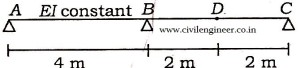8. Analyse the given beam using matrix method :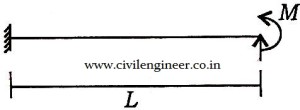9. A Continuous beam of two equal spans L is uniformly loaded over its entire length. Find the magnitude R of the middle reaction using the Castigliano’s theorem :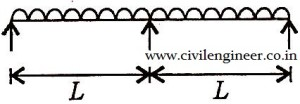10.  A continuous beam ABC is loaded as shown in fig.Determine all reactions and draw BMD and SFD.